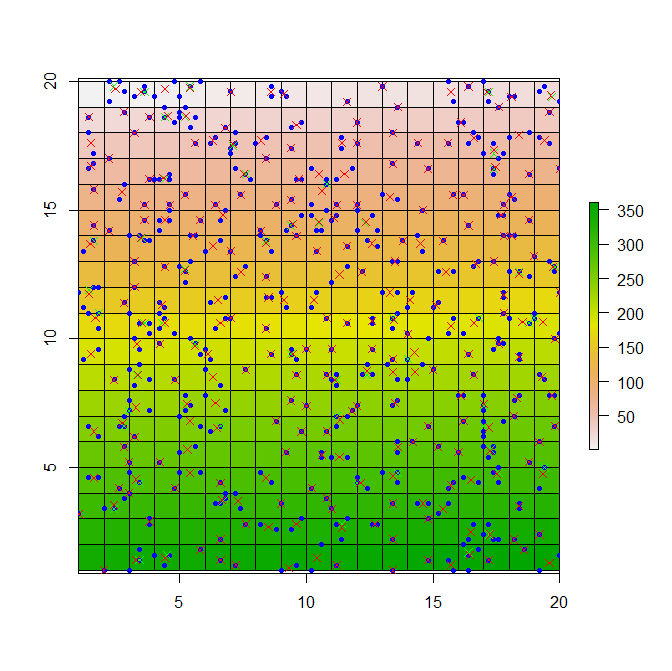# Replicating GMT's blockmedian/blockmean in R

I have frequently used GMT’s blockmean and blockmedian functions with great success. These method essentially quasi-grid your points which is a particularly useful technique when trying to reduce irregularly spaced data such as for onward point sampling or data reduction. One case I have employed this is where the points were to be used as an input to kriging.

Sometimes I need to make use of these blockmean/blockmedian functions within R when system commands cannot be executed. Consequently, I’ve looked for a way to mimic the GMT functions within R - below is my current effort. This could be improved but provides a useful start if you’ve been looking for something similar… data with columns other than x and y are not handled in the below example.

The below code:

2. creates a grid over which the points lie of a given dimension
3. assigns unique ID to each cell (in this case, the cell number)
4. extracts raster cell value to all points within a cell
5. calculates new point positions for all points within a specific cell
6. output new point list - blockmean or blockmedian filtered :)
``````library(sp)
library(raster)

# Create some points
xs=sample(seq(from=1, to=20, by=0.2), size=500, replace=TRUE)
ys=sample(seq(from=1, to=20, by=0.2), size=500, replace=TRUE)
plot(xs,ys)

pnts=SpatialPoints(cbind(xs,ys))
ext=extent(pnts)

# Create grid
nrows=ext-ext
ncols=ext-ext
r = raster(nrows = nrows, ncols = ncols, xmn = ext, xmx = ext, ymn = ext, ymx = ext)

# Assign cell number to each cell
vals=1:ncell(r)
r[]=vals

# Extract cell value to points
pnts\$cell=extract(r, pnts)

# Calculate median point position
# Assumes dataframe with columns x,y,cell (<<< cell number)
# method - median or mean - otherwise fail
block_xy <-function(df, method='median'){

cells=unique(df\$cell)
xys=data.frame()
for (cell_inst in cells){
grp=subset(df, cell==cell_inst)

if (method=='median'){
xs=median(grp\$x)
ys=median(grp\$y)
} else if (method=='mean'){
xs=mean(grp\$x)
ys=mean(grp\$y)
}

xys=rbind(xys,c(xs, ys, cell_inst))
}

colnames(xys)<-c('x','y','cell')
return(xys)
}

# Run the filtering
pnts=as.data.frame(pnts) # cell, xs, ys
colnames(pnts)=c('cell','x','y')
med_xys=block_xy(pnts, method='median')
mean_xys=block_xy(pnts, method='mean')

# Quick plot
plot(r)Quasi-gridded points. Raster is coloured by cell number, original points are in blue, blockmedian points are in green and blockmean points are in red.